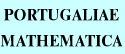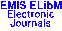PORTUGALIAEMATHEMATICA Vol. 56, No. 3, pp. 265-272 (1999)

Previous Article

Next Article

Contents of this Issue

Other Issues

ELibM Journals

ELibM Home

EMIS Home

## Notes on Galois Extensions with Inner Galois Groups

### Xiao-Long Jiang and George Szeto

Mathematics Department, Zhongshan University,
510275 Guangzhou - P. R. CHINA
Peoria, Illinois, 61625 - U.S.A.

Abstract: Let \$S\$ be a ring with 1, \$C\$ the center of \$S\$, \$G\$ a finite inner automorphism group of \$S\$ of order \$n\$ for some integer \$n\$ invertible in \$S\$ where \$G=\{g_{1},g_{2},...,g_{n}\}\$ and \$g_{i}(s)=U_{i}\,s\,U_{i}^{-1}\$ for some \$U_{i}\$ in \$S\$ and all \$s\$ in \$S\$, and \$R\$ the subring of all elements fixed under each element in \$G\$. Then, \$S\$ is a \$G\$-Galois extension of \$R\$ which is an Azumaya \$C\$-algebra with a Galois system \$\{n^{-1}U_{i},\,U_{i}^{-1}\}\$ if and only if \$S\$ is a projective group ring \$RG_{f}\$ for some factor set \$f\$ which is an \$H\$-separable extension of \$R\$ and \$R\$ is a separable \$C\$-algebra. Moreover, some correspondence relations are given between certain sets of separable subalgebras of such an \$S\$.

Keywords: Galois extensions; projective group rings; Azumaya algebras; \$H\$-separable extensions.

Classification (MSC2000): 16S30, 16W20.

Full text of the article: